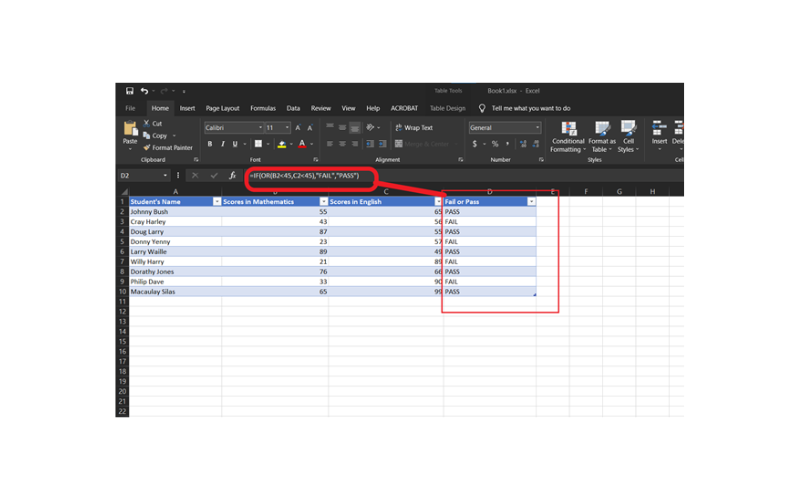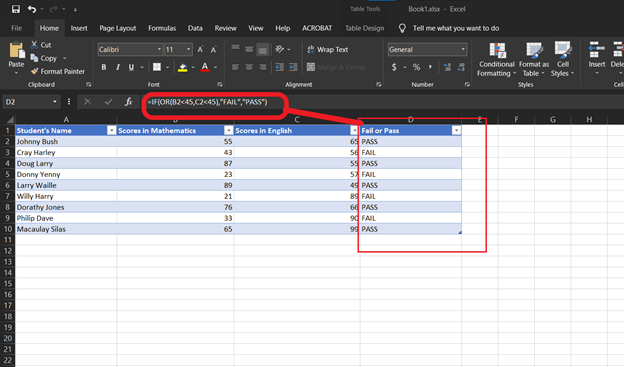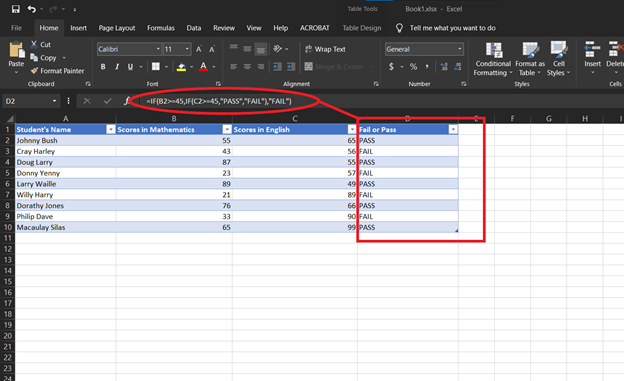# Excel IF between Multiple RangesOver the years, excel has helped many with the task of proper and effortless data representation and organization.

Different firms, industries, and individuals have harnessed the various tools on excel and applied them to their dataset to have their data in the best form possible and to do a proper analysis of their data.

Just as mentioned earlier, excel is a package that houses many great functions that are useful and largely instrumental in the analysis and organization of data, and one of these very useful functions is the IF function.

The IF function is a very useful function and also one of the most popular functions in excel and it’s majorly useful for testing something in our dataset and arriving at a conclusion if it is true or false. As a result, an IF statement can have two outcomes.

If your comparison is true, the first result is true; if your comparison is false, the second result is false.

The IF function is a great statistical tool, especially when dealing with probabilities in mathematics.

It has great use in statistics as it helps to give an estimate of logic that are true and those that are false.

In this article, we shall be looking at how to use Excel IF between multiple ranges.

Before we go into it in detail, however, I would like to show us some very salient benefits/ advantages of the IF function in excel.

• It does a simple logic test (is a sentence TRUE or FALSE?) and returns one value if the result is TRUE, and another value if the result is FALSE, based on the comparison result.
• When working with large data, it helps to ease stress and get comparisons between the dataset done quickly.
• It helps to make conclusions and generalizations about the dataset being analyzed.
• This function in excel is particularly useful for teachers who have quite a lot of data to input and come to a logical conclusion over and so it’s safe to call this function the tutor’s companion.

I hope you have been able from the above-outlined usefulness of this wonderful function able to know more about its application to the dataset.

Now let’s go a bit further and dive into what it is all about and how to incorporate it into the set of data we have to work with to get the best out of this function.

## Components of the IF function

Majorly, there are three components of the IF function and these are what make up the IF function.

1. A logical test that compares two numbers using an operator sign;
2. There will be a value to return from the dataset if the logic test is TRUE
3. There will be a value to return from the dataset if the logic test is FALSE

Now let’s consider the various ways by which we can apply the IF function to our dataset in excel.

We shall be considering four different ways by which we can achieve this.

1. For OR Type Criteria between Multiple Ranges, Excel’s IF and OR Functions can be used.

To handle an OR type condition between several ranges, you can use a combination of Excel’s IF function and the OR function.

Let’s take an example of a certain set of students and consider their scores in mathematics and English and then check to see if each student has passed or failed the subject.

In this example, the condition that must be fulfilled to warrant a student failing is that he/she scores below 45.

So if a student fails one of the two subjects, the student has failed.

Considering the conditions stated above to be used in this example, we see that it’s a 0R type condition between multiple ranges.

`=IF(OR(B2<45,C2<45),”FAIL”,”PASS”)`

We then copy this formula to the rest of the cells by dragging the fill handle.From the results being displayed after applying the formula to our dataset, we see that students who failed one of the subjects by scoring below 45 were tagged as FAIL.

Now, let me give a little exegesis of the formula that gave birth to the results as displayed:

• The role of B2<45 was to send back TRUE as feedback once the score in cell B2 under mathematics was less than 45 and to return FALSE if the score is greater than 45. The same applies to C2 (score for English).
• The role of OR(B2<45,C2<45) was to send back TRUE as feedback if one of the values between B2 and C2 was less than 45 and to return FALSE if the values between B2 and C2 was more than 45.
• And then, the role of IF(OR(B2<45,C2<45),”FAIL”,”PASS”) was to send back FAIL if it receives TRUE from the function aforementioned and otherwise to send PASS
1. For AND Type criteria between multiple ranges, Excel’s IF and AND functions can be used.

In excel, the IF and AND functions can be combined for the AND type criteria between multiple ranges.

So from our already cited example, let’s try to decide whether the students passed or not using the AND function together with IF.

`=IF(AND(B2>=45,C2>=45),”PASS”,”FAIL”)`

We then copy this formula to the rest of the cells by dragging the fill handle.Let me also give a little exegesis of the formula that gave birth to the results as displayed:

• The role of B2>=45 was to send back TRUE as feedback once the score in cell B2 under mathematics was less than 45 and to return FALSE if the score is greater than 45. The same applies to C2 (score for English).
• The role of AND(B2>=45,C2>=45) was to send back TRUE as feedback if one of the values between B2 and C2 was equal to or greater than 45 and otherwise FALSE.
• And then, the role of IF(AND(B2>=45,C2>=45),”PASS”,”FAIL”) was to send back PASS if it receives TRUE from the function aforementioned and otherwise to send FAIL.
1. For AND Type criteria between multiple ranges, Excel’s Nested IF Function can be used.

In excel, the nested IF function can be used to handle a AND type condition between several ranges.

Let’s consider the example we have been using and apply this method to it.

`=IF(B2>=45,IF(C2>=45,”PASS”,”FAIL”),”FAIL”)`

We then copy this formula to the rest of the cells by dragging the fill handle.Let me also explain the way this formula works:

• If B2>=45 is TRUE, the formula enters IF(C2>45,”PASS”,”FAIL”); otherwise, “FAIL” is returned.
• Also if C2>=45 is TRUE, then it returns “PASS” as feedback and otherwise returns “FAIL”.
• As a result, it only returns “PASS” if both subjects are passed; otherwise, it returns “FAIL”.
1. For AND Type Criteria between Multiple Ranges, Excel’s IFS function can be used

Also in excel, we can harness the IFS function to handle a AND type condition between several ranges.

Still considering the example that has already been cited, let’s go on to see how the IFS function can be applied to getting our desired result.

`=IFS(B2<45,"Fail",C2<45,"Fail",TRUE,"Pass")`Let me explain this last method also:

• With the first TRUE parameter, the IFS function sends back the equivalent value; otherwise, a N/A error is returned.
• If the value is B2<45, the result is “FAIL”. If not, it checks whether C2<45 is present. If this is the case, it will return “FAIL”.
• If the C2<45 is also FALSE, the next TRUE is encountered, and the result is “PASS”.

And then, we have our desired result.

## Conclusion

So far, we have considered various ways to use the IF function and also looked at other functions that go along with it in getting the desired result and we have also considered the fact that this function is a logical tool that helps to make reasonable comparisons and arrive at a conclusion of a particular part of the dataset being TRUE or FALSE.

Making the most of this piece is dependent on your attentiveness to detail.

The various methods of getting results with this function have been carefully outlined and explained in this material.

If during the process of following this guide you are stuck anywhere, go back to the beginning and read it carefully and also take note of the steps thoroughly.

It is good to take note of the fact that though excel is not case sensitive, yet, the commands for the functions will not be executed if there is a mistake when inputting the formula.

If the symbols are not correctly placed, the formula will not execute the command and so it is important to check up well on the symbols used in the formulas.

Take note that the brackets used are () and not [].

Since excel is a program, the possibility of it making a mistake is almost zero and so errors in the use of this function will most likely be from the user.

I hope you found this piece enlightening and helpful.

Ovo is a highly specialized Computer and Networking Expert with experience in Windows, Cisco, Microsoft Office (Excel, Word, etc) and Networking engineering. Ovo is a creative, team player that loves sharing his experience with technology with readers to follow along. He has great attention to detail when discussing various technologies, tutorials and guides.